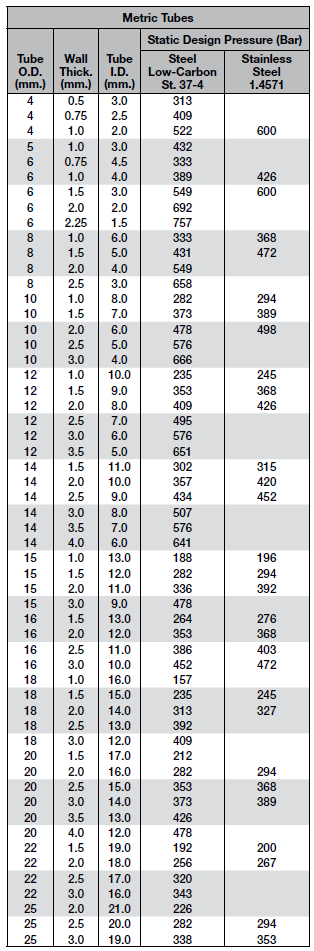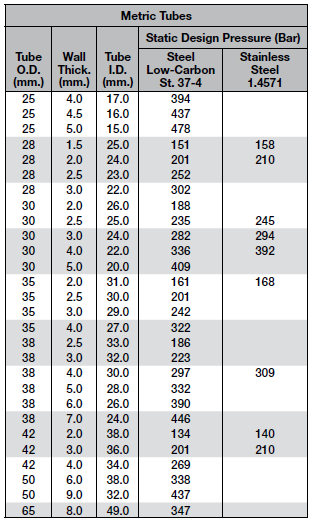# Pressure Rating for Metal Tubing

Pressure ratings for metal tubing shown here are for dynamic systems. A vast majority of systems where our fittings are used fall in this category. However, there are applications, such as hydraulic jacks, where the system pressure is essentially static once it is pressurized. For this type of an application, Parker allows the fittings to be used at higher pressures.

## Dynamic Hydraulic System

A system in which the operating pressure fluctuates, in accordance with load, up to a maximum pressure limited by the relief valve. In addition, the system may also experience shocks, vibration and temperature excursions.
Example: A backhoe.

## Static Hydraulic Systems

A system that, once pressurized, is essentially free of pressure fluctuations, shock, vibration and temperature excursions, with such pressurizations not exceeding 30,000 in the life of the system.
Example: A hydraulic jack.

The dynamic pressure ratings are based on a minimum design factor of 4. In other words, the fitting is capable of holding a pressure equal to 4 times the rated pressure before leakage or failure. For static applications, the design factor can lowered to 3, so the static pressure rating is 1/3 higher than the dynamic pressure rating.

STATIC PRESSURE RATING = DYNAMIC PRESSURE RATING X 1.33

Example: Static pressure for a fitting rated to 3,000 PSI is 4,000 PSI (3,000 x 1.33 = 4,000).

Some Parker fittings and adapters are capable of performing at a higher pressure than their published pressure ratings. For information about higher pressure ratings, contact Parker Hannifin Tube Fittings Division or Motion & Flow Control Products, Inc.

The dynamic pressure rating for tubing is calculated using LAME's Design Pressure Formula: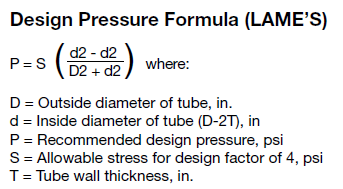For thin wall tubing (D/T = 10 or more), use the following formula: P = 2ST/DLAME's formula was used to calculate values in the pressure rating tables for inch and metric tubing provided below.

## Tubing Selection

1. Consult the Severity of Design Derating Factors table to determine if the pressure rating needs to be derated.
2. Consult the Temperature Derating Factors table to determine if the pressure rating needs to be derated.
3. Calculate the pressure value (X) to look for in the table: X = (Recommended Desing Pressure)/(Severity of Service Factor)(Temperature Factor)
Use this value as your corrected recommended design pressure
4. Using the Inch Pressure Rating and Metric Pressure Rating tables below, determine the tube O.D. and wall thickness combination that has (corrected) recommended design pressure equal to or higher than maximum operating pressure AND tube I.D. is equal to or greater than required flow diameter.

### Severity of Design Derating Factors

If any of the factors in the table below apply to your situation, multiply the maximum working pressure from the pressure rating tables above by the corresponding factor to arrive at the corrected maximum working pressure.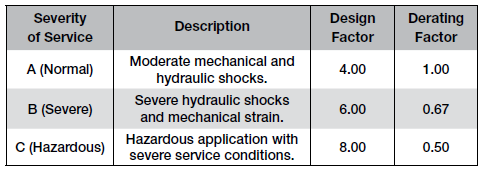### Temperature Derating Factors

If the maximum working temperature of your system is above 100°F, use the table below to determine the derating factor.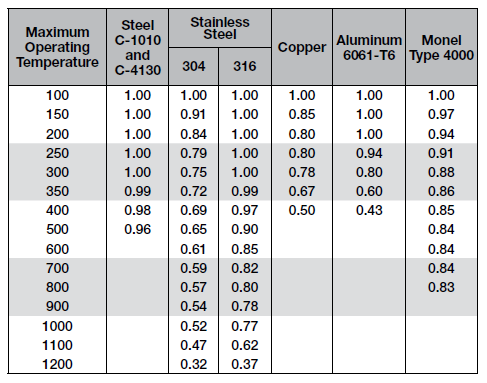### Pressure Rating for Inch Tubing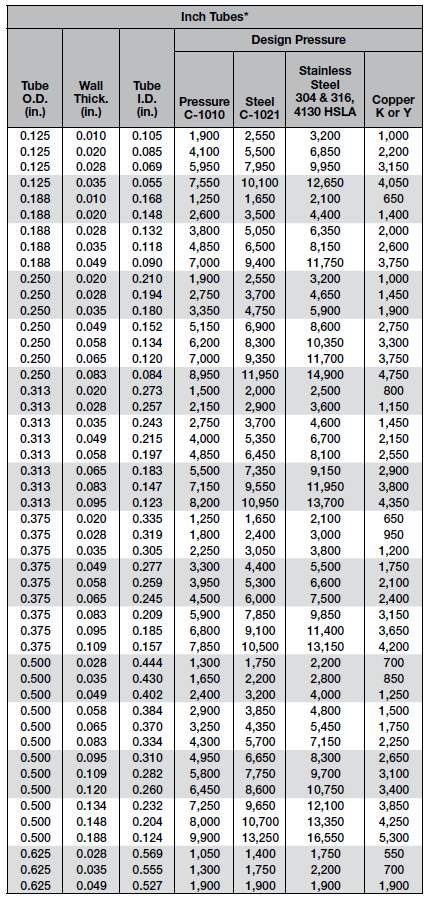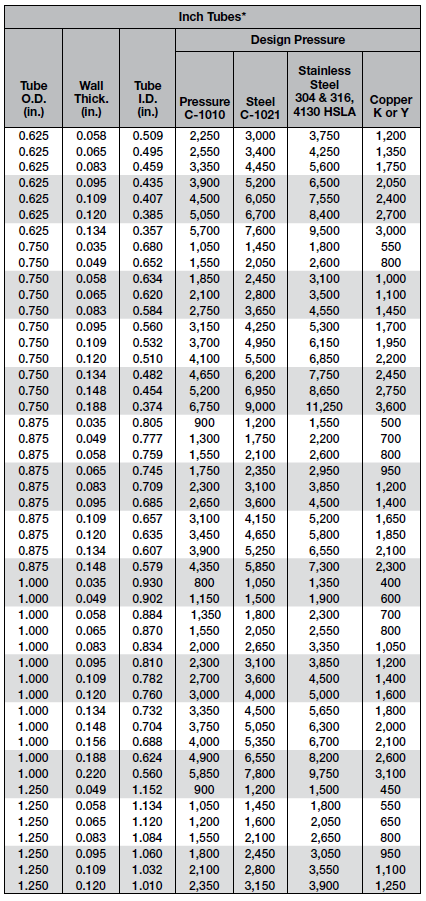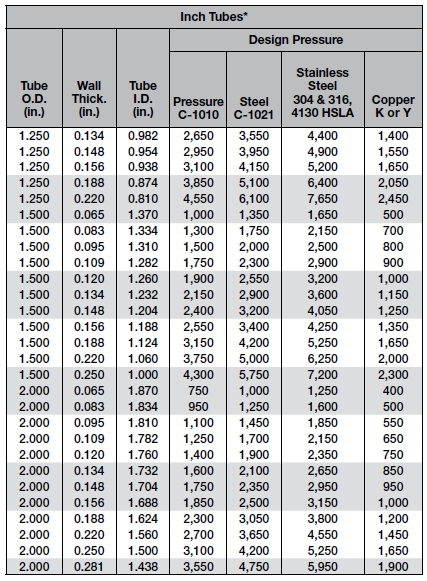### Pressure Rating for Metric Tubing